# Mathematical Logic 2015 Propositional Logic exercises Fausto Giunchiglia

• Slides: 11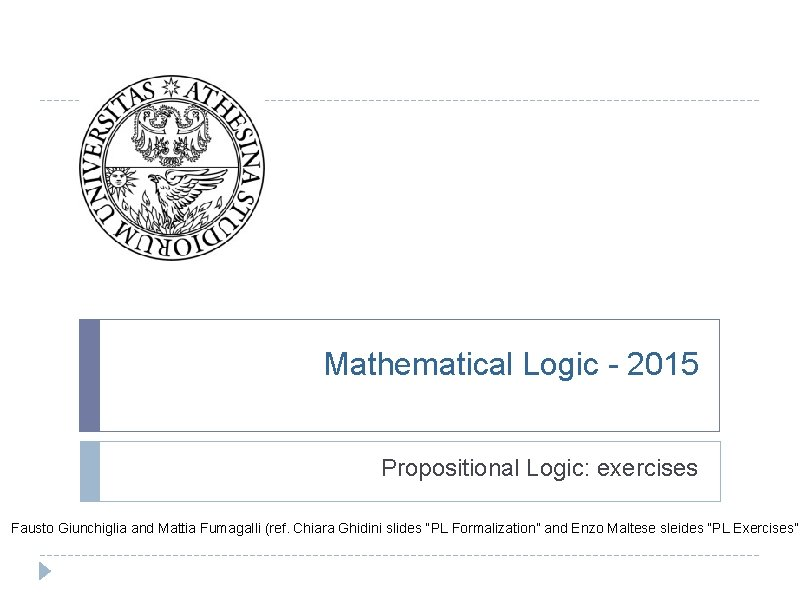Mathematical Logic - 2015 Propositional Logic: exercises Fausto Giunchiglia and Mattia Fumagalli (ref. Chiara Ghidini slides “PL Formalization” and Enzo Maltese sleides “PL Exercises”)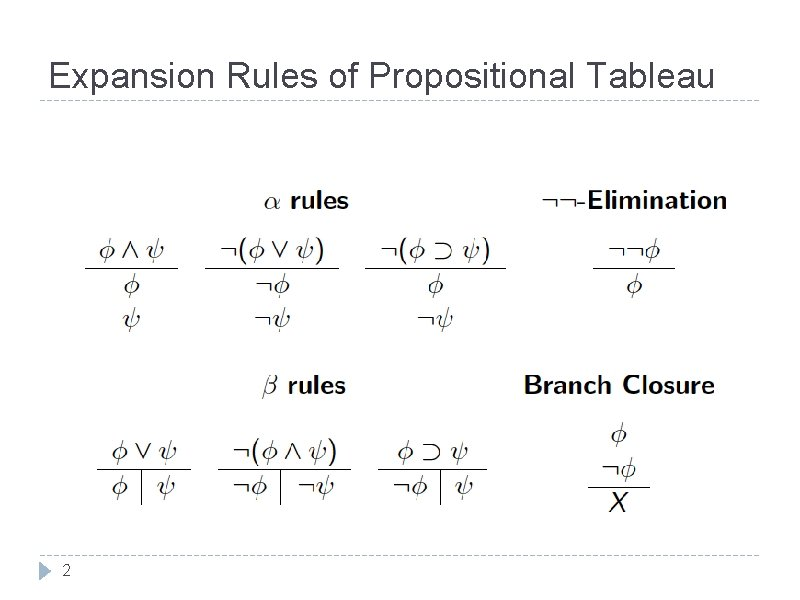Expansion Rules of Propositional Tableau 2Tableaux: exercise 1 Is the following formula satisfiable? ((P Q) P) (P Q) P P P Q X 3Tableaux: Exercise 2 Is the following formula valid? (and satisifiable? ) ((P Q) P) (P Q) P P X 4 QTableaux: Exercise 3 Is the following formula satisifable? ((P Q) (P Q R) (P R)) (P Q) (P Q R) (P Q) (P Q R) P R P Q (P Q) X P X 5 Q X R X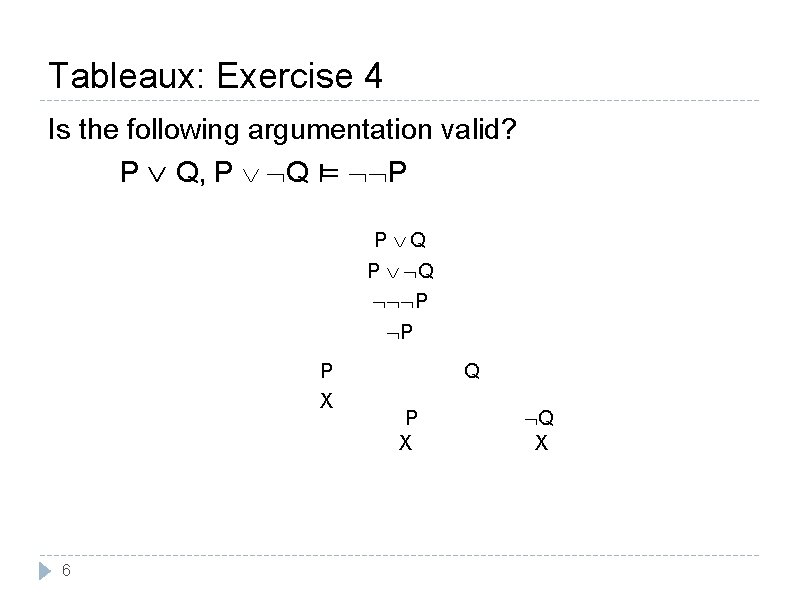Tableaux: Exercise 4 Is the following argumentation valid? P Q, P Q ⊨ P P Q P Q P P P X 6 Q P X Q X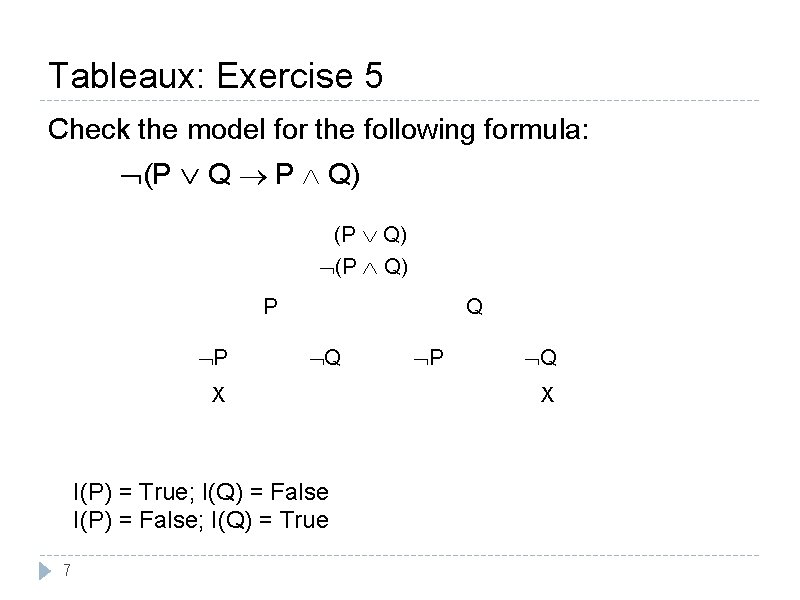Tableaux: Exercise 5 Check the model for the following formula: (P Q P Q) (P Q) P P Q Q X I(P) = True; I(Q) = False I(P) = False; I(Q) = True 7 P Q X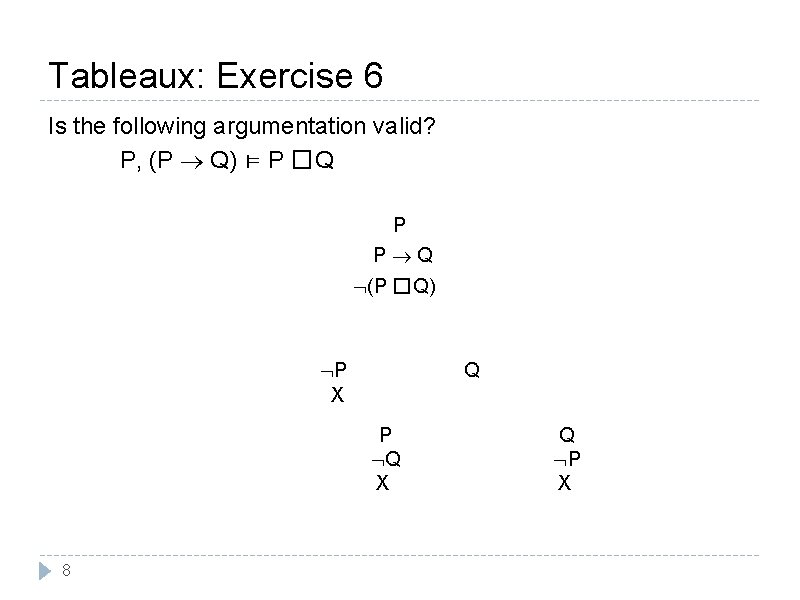Tableaux: Exercise 6 Is the following argumentation valid? P, (P Q) ⊨ P �Q P P Q (P �Q) P X Q P Q X 8 Q P X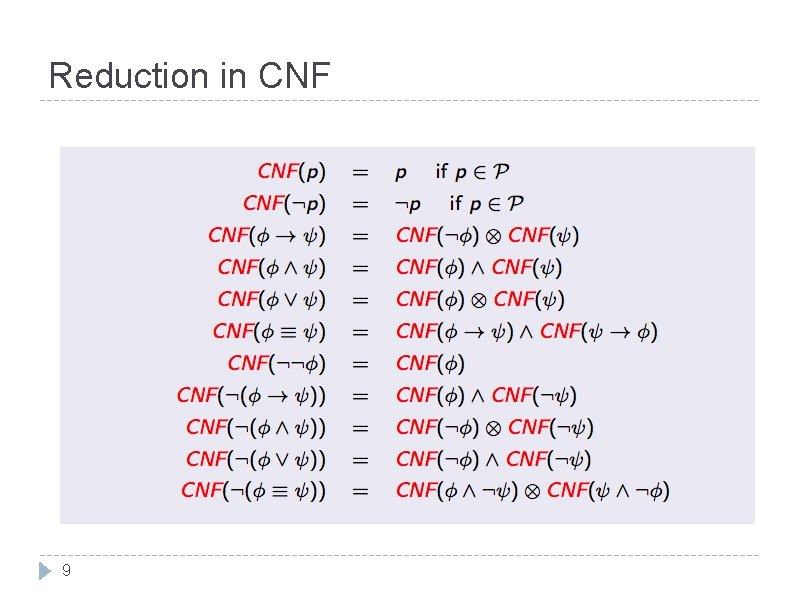Reduction in CNF 9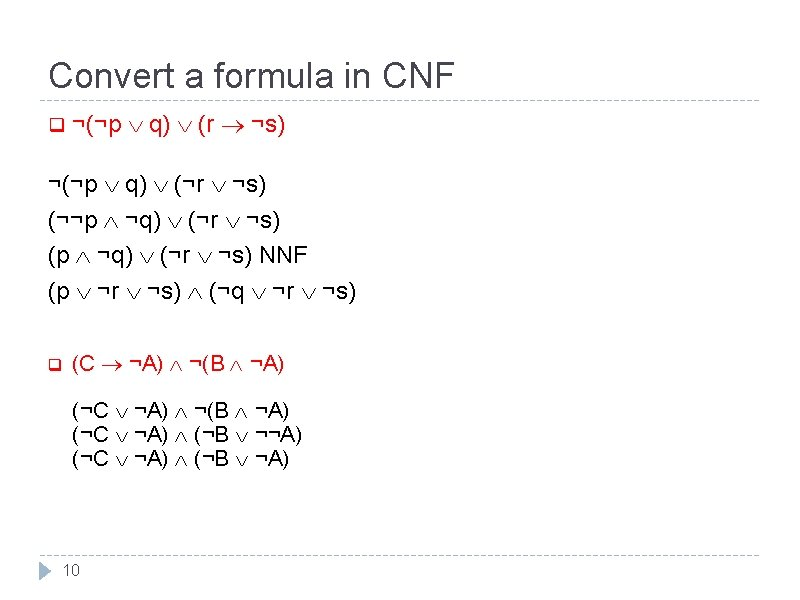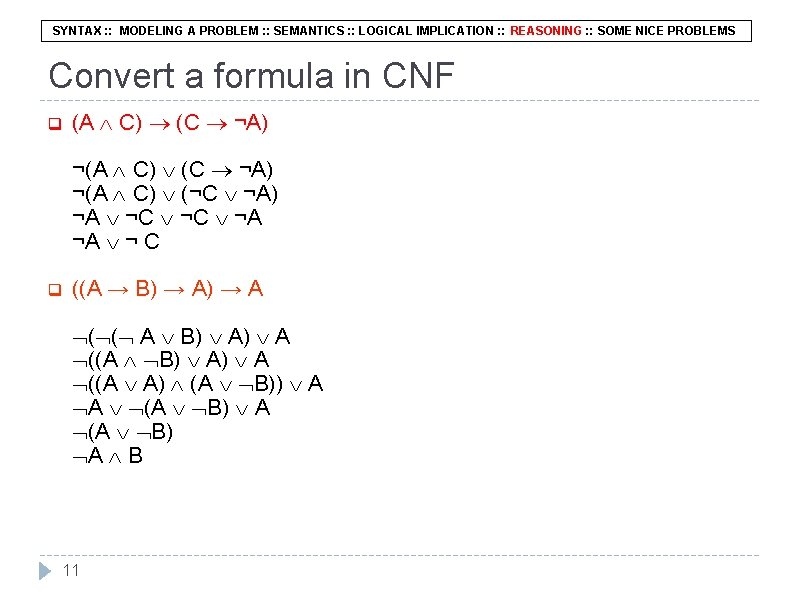SYNTAX : : MODELING A PROBLEM : : SEMANTICS : : LOGICAL IMPLICATION : : REASONING : : SOME NICE PROBLEMS Convert a formula in CNF q (A C) (C ¬A) ¬(A C) (¬C ¬A) ¬A ¬C ¬A ¬A ¬ C q ((A → B) → A ( ( A B) A) A ((A A) (A B)) A A (A B) A (A B) A B 11